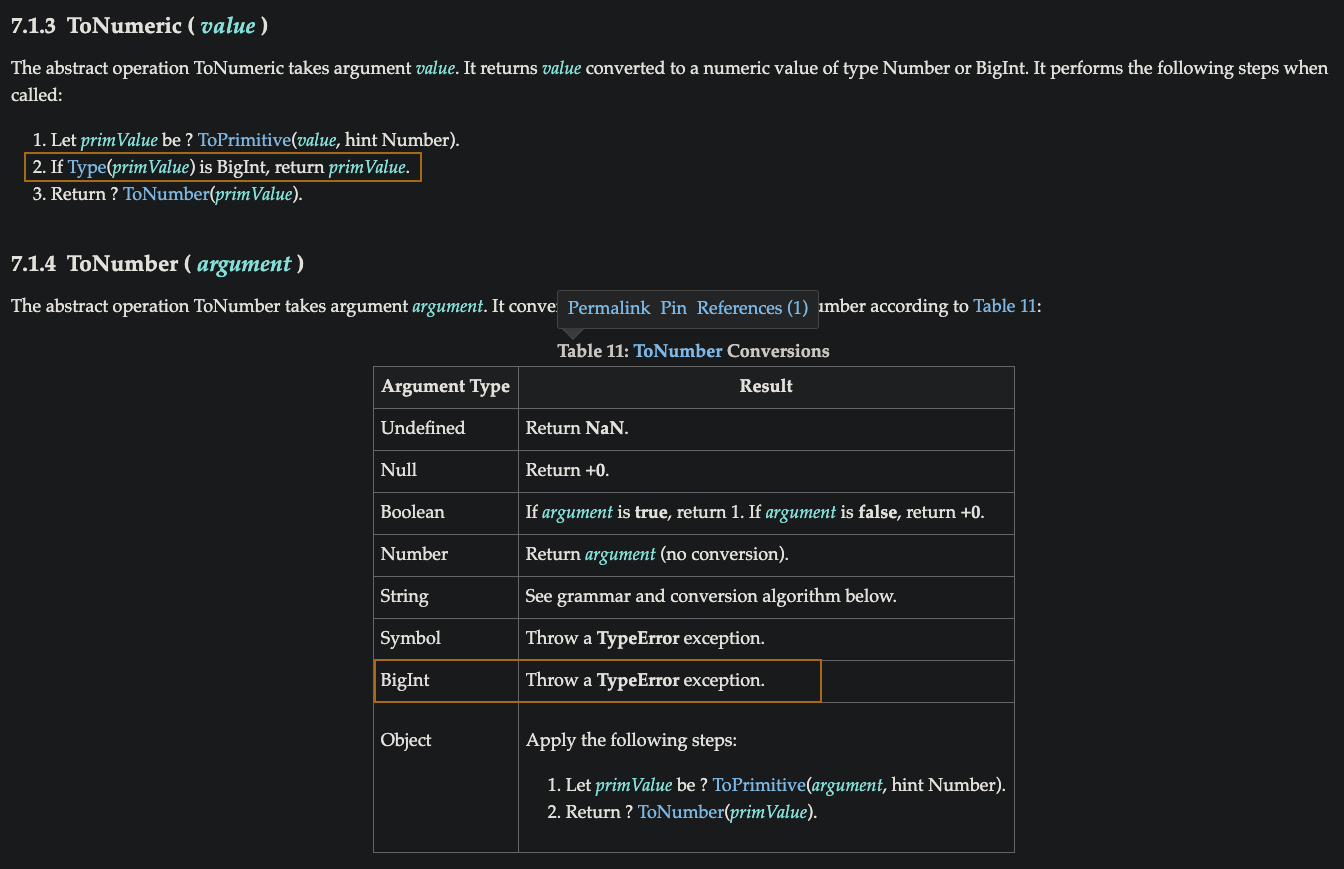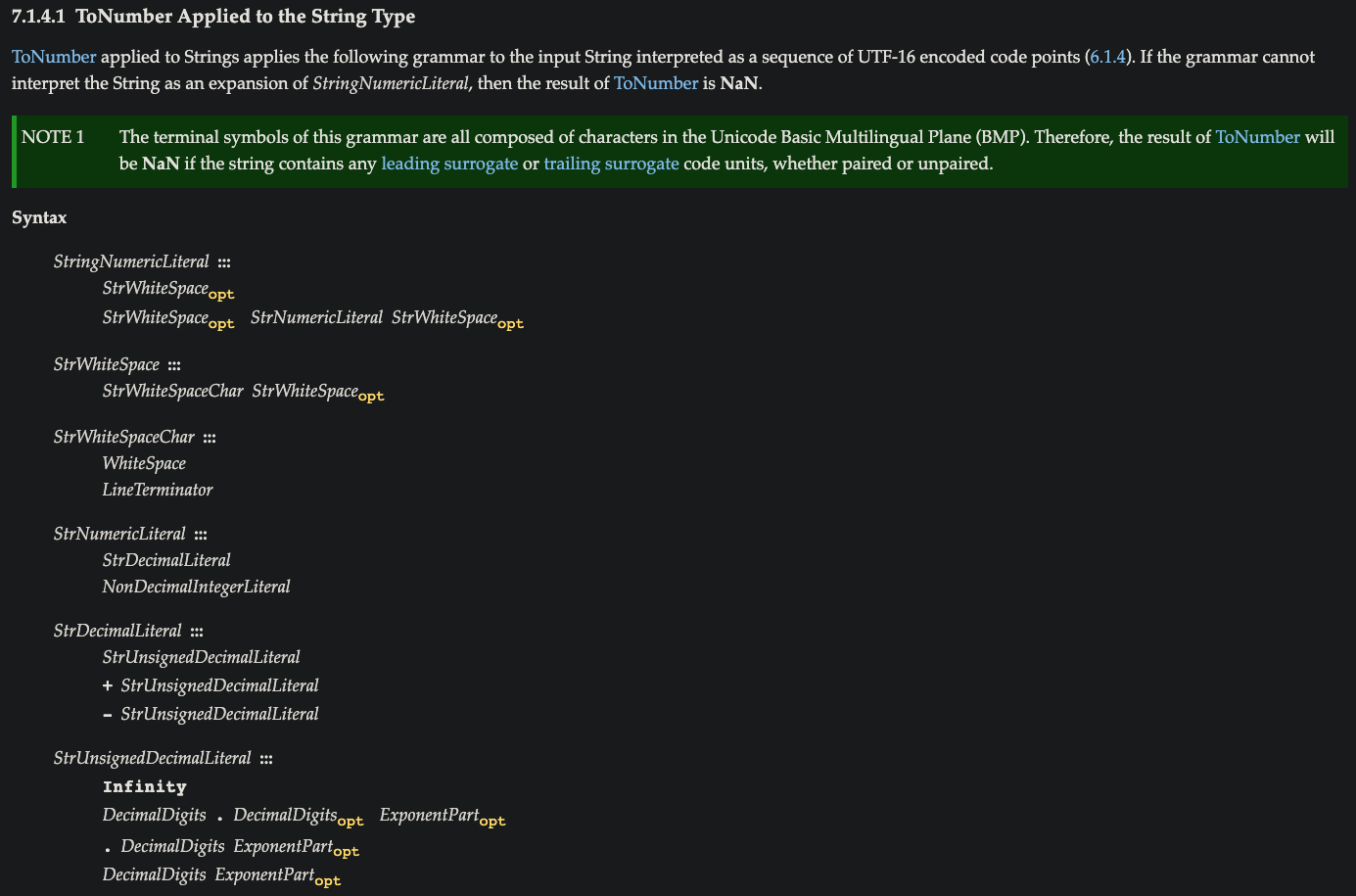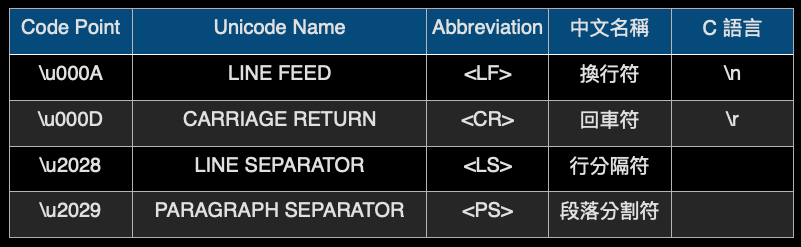#  強制轉型 - ToNumber、ToPrimitive、StringNumericLiteral、NonDecimalIntegerLiteral

keywords:`ToNumber`,`ToPrimitive`,`StringNumericLiteral`,`NonDecimalIntegerLiteral`

### ToNumber

🚩 會特別把 7.1.3 也列出來是因為第二點，如果是 BigInt 回傳其原生值，但是 Table 11 的 BigInt 卻寫擲出一個 TypeError，不過嘗試使用 Number() 或 parseInt() 得到的結果都是其原生值``````    Number(undefined); // NaN

Number(null); // 0

Number(true); // 1

Number(false); // +0   這也呼應先前介紹的 Falsy 值，稍後也會再次提到

Number(Symbol('abc')); // Uncaught TypeError: Cannot convert a Symbol value to a number at Number

Number(1n); // 1

parseInt(1n); // 1
``````

#### String to Number

Table 11 寫 String 轉為 Number 根據下方文法及轉換演算法• StringNumericLiteral 屬於字串的數字 ex: '99'

• StrWhiteSpace ( opt ) 字串的空格符 ( 選擇性的 )

• WhiteSpace 空白

``````  Number(' '); // 0

Number(''); // 0

Number('    '); // 0    tab
``````
• LineTerminator 行終止符``````  Number('\u000A'); // 0

Number('\u000D'); // 0

Number('\u2028'); // 0

Number('\u2029'); // 0

Number('\n'); // 0
``````
• StrNumericLiteral 數字字面值

• StrDecimalLiteral 十進制數

• StrUnsignedDecimalLiteral 無負號的十進制數
• Infinity 無限
• DecimalDigits.DecimalDigits(opt) ExponentPart(opt) 十進制數或帶有( 小數位及指數位 )
• .DecimalDigits ExponentPart(opt) 小數位或帶有（ 指數位 )
• DecimalDigits ExponentPart(opt) 十進制數或帶有（ 指數位 ）
• +StrUnsignedDecimalLiteral 正數無負號的十進制數
• -StrUnsignedDecimalLiteral 負數無負號的十進制數

``````  Number('Infinity'); // Infinity

Number('-Infinity'); // -Infinity

Number('1.2e5'); // 120000

Number('-1.2e5'); // -120000

Number('0.2e5'); // 20000

Number('-0.2e5'); // -20000

Number('1e5'); // 100000

Number('-1e5'); // -100000
``````
• NonDecimalIntegerLiteral 非十進制整數字面值，任何進制轉換成十進制的方法都一樣，有興趣的可以參考這篇文章

• BinaryIntegerLiteral 二進制數

``````  Number('0b101'); // 5

0b 表示為二進制開頭

( 1 * 2^2 ) + ( 0 * 2^1 ) + ( 1 * 2^0 )

= 4 + 0 + 1

= 5
``````
• OctalIntegerLiteral 八進制數

``````  Number('0o127'); // 87

0o 表示為八進制開頭

( 1 * 8^2 ) + ( 2 * 8^1 ) + ( 7 * 8^0 )

= 64 + 16 + 7

= 87
``````
• HexIntegerLiteral 十六進制數

``````  Number('0x1FB'); // 507

0x 表示為十六進制開頭

------------------------------------------
1 2 3 4 5 6 7 8 9 10 11 12 13 14 15 -> 十進制

1 2 3 4 5 6 7 8 9 A  B  C  D  E  F  -> 十六進制
------------------------------------------

( 1 * 16^2 ) + ( 15 * 16^1 ) + ( 11 * 16^0 )

= 256 + 240 + 11

= 507
``````

1. 字串的數字可以包含開頭或結尾為 空白或行終止符
2. 字串的數字十進制可以包含 0 為開頭的任何數字
3. 字串的數字可以包含一個 + - 符號代表他的正負值
4. 字串的數字如果是 empty 或空白會回傳 +0
5. Infinity -Infinity 被識別為字串的數字，而不能識別為數字字面值
6. 字串的數字不能是 BigInt

`````` Number('\u000A15'); // 15

Number('\u000A 1.5\u000D'); // 1.5

Number(' 15'); // 15

Number(' 1 5 '); // NaN  上方 1. 只能包含開頭或結尾為空白，所以空白放在 1 和 5 之間就不行

Number('015'); // 15

Number('-15'); // -15

Number(''); // 0

Number(' '); // 0

Number('1n'); // NaN
``````

#### Object to Number

step 1. 物件型別值會先被轉為基本型別值等效值，為了轉換基本型別值，使用 ToPrimitive 抽象運算
step 2. 會查看是否具有 valueOf()，如果有就調用，假設回傳一個基本型別值，那個值就會被強制轉型為數字
step 3. 如果 valueOf() 回傳的依舊是物件，但還有 toString() 就會提供用於強制轉型的值
step 4. 如果 valueOf()、toString() 都回傳非基本型別值就擲出一個 TypeError

``````    let c = [9,8];

c.toString = function(){

return this.join('');

};

Number(c); // 98

// 這時增加優先順序高於 toString() 的 valueOf()
c.valueOf = function() {

return this.join('.');

};

Number(c); // 9.8
``````

1.`Number([]); // 0`

``````    根據上方 step 2

[].valueOf(); // []  回傳還是一個 [] 並非基本型別值，所以查看是否有 toString() 方法

根據上方 step 3

[].toString(); // "" 在上面補充的 4.中提到輸入的字串如果是 empty 或空白會回傳 +0

∴ Number(""); // 0
``````

2.`Number({}); // NaN`

``````    ({}).valueOf(); // {}  回傳 {} 尚不是基本型別值，故查看是否有 toString() 方法

({}).toString(); // "[object Object]"  這是一個基本型別值 String 所以提供給 Number 做數字轉型

Number("[object Object]"); // NaN  參考下方說明

P.S. {} 要用 () 包起來，不然程式會以為 {} 是一個 block
``````

If the grammar cannot interpret the String as an expansion of StringNumericLiteral, then the result of ToNumber is NaN.

3.`Number(['abc']); // NaN`

``````    ['abc'].valueOf(); // ["abc"]  回傳依然是一個陣列，尚不是基本型別值，故查看是否有 toString() 方法

["abc"].toString(); // "abc"  這是一個基本型別值 String 所以提供給 Number 做數字轉型

Number("abc"); // NaN  如同例 2
``````

#ToNumber #Toprimitive #StringNumericLiteral #NonDecimalIntegerLiteral